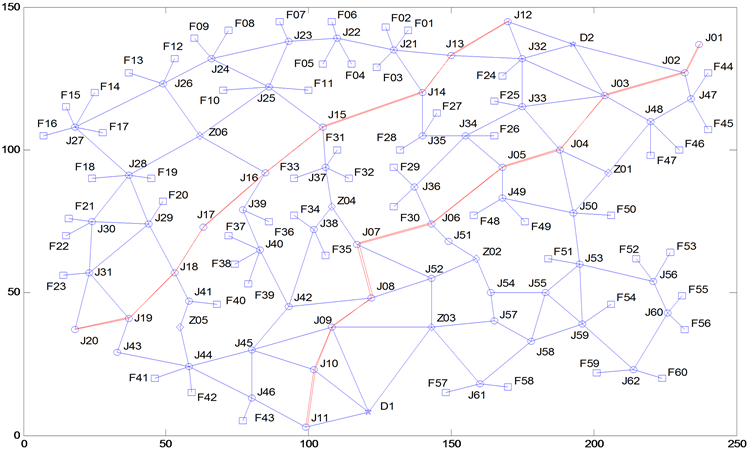# 规划多波次导弹发射Planning in Multi Wave Missile Launching

DOI: 10.12677/MOS.2021.101013, PDF, HTML, XML, 下载: 28  浏览: 62

Abstract: Missiles play an important role in military operations, so it is very important to make mobile routes. In this problem, the node conflict, concealment, and time limit at the transfer point should be fully considered. At the same time, the temporary increase of transfer area should be considered. It is a combinatorial optimization problem to plan the travel time and route of each missile launcher. Based on this problem, a global ergodic search algorithm based on Dijkstra is proposed. Taking the shortest total exposure time as the objective function, a multi wave missile launch optimization model is established. The results show that the research results can provide effective engineering application guidance for missile launch time planning.

1. 引言

2. 多波次导弹发射中的规划模型

2.1. 模型建立

$T={T}_{l}+{T}_{w}-{T}_{s}-{T}_{z}$ (1)

${T}_{l}=\underset{k}{\sum }\underset{i}{\sum }\underset{l=1}{\overset{{n}_{k,i-1}}{\sum }}{t}_{{p}_{k,l}^{i}{p}_{k,l+1}^{i}}$

${T}_{w}=\underset{k}{\sum }\underset{i}{\sum }\underset{l=1}{\overset{{n}_{k,i}}{\sum }}{w}_{{p}_{k,l}^{i}}$

${T}_{s}=\underset{k}{\sum }{w}_{k,1}^{1}$

${T}_{z}=\underset{k}{\sum }\left({w}_{k,{n}_{k,2}}^{2}+{w}_{k,1}^{3}\right)$

${s}_{k}^{1}\in \left\{{D}_{1},{D}_{2}\right\}$ (2)

${e}_{k}^{1}\in \left\{{F}_{1},{F}_{2},\cdots ,{F}_{60}\right\}$ (3)

${f}_{{p}_{k,l}^{1},{p}_{k,l+1}^{1}}=1$ (4)

$\left({p}_{{k}_{i,l},}^{1}{p}_{{k}_{i,l+1}}^{1}\right)=-\left({p}_{{k}_{j},m}^{1},{p}_{{k}_{j},m+1}^{1}\right)|\Delta {t}_{{p}_{{k}_{i,l}}^{1}{p}_{{k}_{i,l+1}}^{1}}^{{k}_{i}}\cap \Delta {t}_{{p}_{{k}_{j,m}}^{1},{p}_{{k}_{j,m+1}}^{1}}^{{k}_{j}}=\varphi$ (5)

$\underset{l=1}{\overset{{n}_{k,1-1}}{\sum }}{t}_{{p}_{k,l}^{1},{P}_{k,l+1}^{1}}+\underset{l=1}{\overset{{n}_{k,l}}{\sum }}{w}_{k,l}^{i}={t}_{mission}^{1}$ (6)

$\left\{\begin{array}{l}\Delta {t}_{{p}_{k,l}^{1},{p}_{k,l+1}^{1}}^{k}=\left[{T}_{{p}_{k,l}^{i}}^{k}+{w}_{k,l}^{1},{T}_{{p}_{k,l+1}^{i}}^{k}\right]\\ {T}_{{p}_{k,l}^{i}}^{{k}_{i}}=\underset{m=1}{\overset{l-1}{\sum }}\left({t}_{{p}_{k,m}^{1},{p}_{k,m+1}^{1}}+{w}_{k,m}^{1}\right)\end{array}$ (7)

${s}_{k}^{2}={e}_{k}^{1}$ (8)

${e}_{k}^{2}\in \left\{{Z}_{1},{Z}_{2},\cdots ,{Z}_{6}\right\}$ (9)

${f}_{{p}_{k,l}^{2},{p}_{k,l+1}^{2}}=1$ (10)

$\left\{\left({p}_{{k}_{i},l}^{2},{p}_{{k}_{i},l+1}^{2}\right)=-\left({p}_{{k}_{j},m}^{2},{p}_{{k}_{j},m+1}^{2}\right)|\Delta {t}_{{p}_{k,l}^{2},{p}_{k,l+1}^{2}}^{{k}_{i}}\cap \Delta {t}_{{p}_{{k}_{j},m}^{2},{p}_{{k}_{j},m+1}^{2}}^{{k}_{j}}=\varphi \right\}$ (11)

$\left\{{k}_{i}\ne {k}_{j},{e}_{{k}_{i}}^{2}={e}_{{k}_{i}}^{2}||{T}_{{p}_{{k}_{i,},{n}_{{k}_{i,2}}}^{2}}^{{k}_{i}}-{T}_{{p}_{{k}_{j,},{n}_{{k}_{j,2}}}^{2}}^{{k}_{j}}|\ge 10\right\}$ (12)

${s}_{k}^{3}={e}_{k}^{2}$ (13)

${e}_{k}^{3}\in \left\{{F}_{1},{F}_{2},\cdots ,{F}_{60}\right\},{e}_{k}^{3}\ne {e}_{k}^{1}$ (14)

${f}_{{p}_{k,l}^{3},{p}_{k,l+1}^{3}}=1$ (15)

$\left\{\left({p}_{{k}_{i},l}^{3},{p}_{{k}_{i},l+1}^{3}\right)=-\left({p}_{{k}_{j},m}^{3},{p}_{{k}_{j},m+1}^{3}\right)|\Delta {t}_{{p}_{k,l}^{3},{p}_{k,l+1}^{3}}^{{k}_{i}}\cap \Delta {t}_{{p}_{{k}_{j},m}^{3},{p}_{{k}_{j},m+1}^{3}}^{{k}_{j}}=\varphi \right\}$ (16)

$\left(\underset{l=1}{\overset{{n}_{k,2}-1}{\sum }}{t}_{{p}_{k,l}^{2},{p}_{k,l+1}^{2}}+\underset{l=1}{\overset{{n}_{k,2}}{\sum }}{w}_{k,l}^{2}\right)+\left(\underset{l=1}{\overset{{n}_{k,3}-1}{\sum }}{t}_{{p}_{k,l}^{3},{p}_{k,l+1}^{3}}+\underset{l=1}{\overset{{n}_{k,3}}{\sum }}{w}_{k,l}^{3}\right)={t}_{mission}^{2}$ (17)

$\mathrm{min}{T}^{\prime }=\underset{k}{\sum }\underset{i}{\sum }{\sum }_{l=1}^{{n}_{k,i}-1}{t}_{{p}_{k,l}^{i},{p}_{k,l+1}^{i}}$ (18)

${T}^{\prime }$ ——表示目标函数，简化整体暴露时间；

${t}_{{p}_{k,l}^{i},{p}_{k,l+1}^{i}}$ ——表示第i阶段第k辆车载发射装置经过机动路径中的第l段所耗费的时间， ${t}_{{p}_{k,l}^{i},{p}_{k,l+1}^{i}}=\frac{{d}_{{p}_{k,l}^{i},{p}_{k,l+1}^{i}}}{{v}_{k}}$

$\left\{\begin{array}{l}{s}_{k}^{1}\in \left\{{D}_{1},{D}_{2}\right\},{s}_{k}^{2}={e}_{k}^{1},{s}_{k}^{3}={e}_{k}^{2}\text{\hspace{0.17em}}\text{\hspace{0.17em}}\text{\hspace{0.17em}}\text{\hspace{0.17em}}\text{\hspace{0.17em}}\text{\hspace{0.17em}}\text{\hspace{0.17em}}\text{\hspace{0.17em}}\text{\hspace{0.17em}}\text{\hspace{0.17em}}\text{\hspace{0.17em}}\text{\hspace{0.17em}}\text{\hspace{0.17em}}\text{\hspace{0.17em}}\text{\hspace{0.17em}}\text{\hspace{0.17em}}\text{\hspace{0.17em}}\text{\hspace{0.17em}}\text{\hspace{0.17em}}\text{\hspace{0.17em}}\text{\hspace{0.17em}}\text{\hspace{0.17em}}\text{ }\text{ }\text{\hspace{0.17em}}\text{\hspace{0.17em}}\text{\hspace{0.17em}}\text{\hspace{0.17em}}\text{\hspace{0.17em}}\text{\hspace{0.17em}}\text{\hspace{0.17em}}\text{\hspace{0.17em}}\text{\hspace{0.17em}}\text{\hspace{0.17em}}\text{\hspace{0.17em}}\text{\hspace{0.17em}}\text{\hspace{0.17em}}\left(19\right)\\ {e}_{k}^{1}\in \left\{{F}_{1},{F}_{2},\cdots ,{F}_{60}\right\}\text{\hspace{0.17em}}\text{\hspace{0.17em}}\text{\hspace{0.17em}}\text{\hspace{0.17em}}\text{\hspace{0.17em}}\text{\hspace{0.17em}}\text{\hspace{0.17em}}\text{\hspace{0.17em}}\text{\hspace{0.17em}}\text{\hspace{0.17em}}\text{\hspace{0.17em}}\text{\hspace{0.17em}}\text{\hspace{0.17em}}\text{\hspace{0.17em}}\text{\hspace{0.17em}}\text{\hspace{0.17em}}\text{\hspace{0.17em}}\text{\hspace{0.17em}}\text{\hspace{0.17em}}\text{\hspace{0.17em}}\text{\hspace{0.17em}}\text{\hspace{0.17em}}\text{\hspace{0.17em}}\text{\hspace{0.17em}}\text{\hspace{0.17em}}\text{\hspace{0.17em}}\text{\hspace{0.17em}}\text{\hspace{0.17em}}\text{\hspace{0.17em}}\text{\hspace{0.17em}}\text{\hspace{0.17em}}\text{\hspace{0.17em}}\text{\hspace{0.17em}}\text{\hspace{0.17em}}\text{\hspace{0.17em}}\text{\hspace{0.17em}}\text{\hspace{0.17em}}\text{\hspace{0.17em}}\text{\hspace{0.17em}}\text{\hspace{0.17em}}\text{\hspace{0.17em}}\text{\hspace{0.17em}}\text{\hspace{0.17em}}\text{\hspace{0.17em}}\text{\hspace{0.17em}}\text{\hspace{0.17em}}\text{\hspace{0.17em}}\text{\hspace{0.17em}}\left(20\right)\\ {e}_{k}^{2}\in \left\{{Z}_{1},{Z}_{2},\cdots ,{Z}_{6}\right\}\cup \left\{{J}_{m},{J}_{n}\right\}\text{\hspace{0.17em}}\text{\hspace{0.17em}}\text{\hspace{0.17em}}\text{\hspace{0.17em}}\text{\hspace{0.17em}}\text{\hspace{0.17em}}\text{\hspace{0.17em}}\text{\hspace{0.17em}}\text{\hspace{0.17em}}\text{\hspace{0.17em}}\text{\hspace{0.17em}}\text{\hspace{0.17em}}\text{\hspace{0.17em}}\text{\hspace{0.17em}}\text{\hspace{0.17em}}\text{\hspace{0.17em}}\text{\hspace{0.17em}}\text{\hspace{0.17em}}\text{\hspace{0.17em}}\text{\hspace{0.17em}}\text{\hspace{0.17em}}\text{\hspace{0.17em}}\text{\hspace{0.17em}}\text{\hspace{0.17em}}\text{\hspace{0.17em}}\text{\hspace{0.17em}}\text{\hspace{0.17em}}\text{\hspace{0.17em}}\text{\hspace{0.17em}}\text{\hspace{0.17em}}\text{\hspace{0.17em}}\text{\hspace{0.17em}}\text{\hspace{0.17em}}\text{\hspace{0.17em}}\left(21\right)\\ {e}_{k}^{3}\in \left\{{F}_{1},{F}_{2},\dots ,{F}_{60}\right\},{e}_{k}^{3}\ne {e}_{k}^{1}\text{\hspace{0.17em}}\text{\hspace{0.17em}}\text{\hspace{0.17em}}\text{\hspace{0.17em}}\text{\hspace{0.17em}}\text{\hspace{0.17em}}\text{\hspace{0.17em}}\text{\hspace{0.17em}}\text{\hspace{0.17em}}\text{\hspace{0.17em}}\text{\hspace{0.17em}}\text{\hspace{0.17em}}\text{\hspace{0.17em}}\text{\hspace{0.17em}}\text{\hspace{0.17em}}\text{\hspace{0.17em}}\text{\hspace{0.17em}}\text{\hspace{0.17em}}\text{\hspace{0.17em}}\text{\hspace{0.17em}}\text{\hspace{0.17em}}\text{\hspace{0.17em}}\text{\hspace{0.17em}}\text{\hspace{0.17em}}\text{\hspace{0.17em}}\text{\hspace{0.17em}}\text{\hspace{0.17em}}\text{\hspace{0.17em}}\text{\hspace{0.17em}}\text{\hspace{0.17em}}\text{\hspace{0.17em}}\text{\hspace{0.17em}}\text{\hspace{0.17em}}\text{\hspace{0.17em}}\text{\hspace{0.17em}}\text{\hspace{0.17em}}\text{\hspace{0.17em}}\left(22\right)\\ {f}_{{p}_{k,l}^{i},{p}_{k,l+1}^{i}}=1\text{\hspace{0.17em}}\left(i=1,2,3\right)\text{\hspace{0.17em}}\text{\hspace{0.17em}}\text{\hspace{0.17em}}\text{\hspace{0.17em}}\text{\hspace{0.17em}}\text{\hspace{0.17em}}\text{\hspace{0.17em}}\text{\hspace{0.17em}}\text{\hspace{0.17em}}\text{\hspace{0.17em}}\text{\hspace{0.17em}}\text{\hspace{0.17em}}\text{\hspace{0.17em}}\text{\hspace{0.17em}}\text{\hspace{0.17em}}\text{\hspace{0.17em}}\text{\hspace{0.17em}}\text{\hspace{0.17em}}\text{\hspace{0.17em}}\text{\hspace{0.17em}}\text{\hspace{0.17em}}\text{\hspace{0.17em}}\text{\hspace{0.17em}}\text{\hspace{0.17em}}\text{\hspace{0.17em}}\text{\hspace{0.17em}}\text{\hspace{0.17em}}\text{\hspace{0.17em}}\text{\hspace{0.17em}}\text{\hspace{0.17em}}\text{\hspace{0.17em}}\text{\hspace{0.17em}}\text{\hspace{0.17em}}\text{\hspace{0.17em}}\text{\hspace{0.17em}}\text{\hspace{0.17em}}\text{\hspace{0.17em}}\text{\hspace{0.17em}}\text{\hspace{0.17em}}\text{\hspace{0.17em}}\text{\hspace{0.17em}}\text{\hspace{0.17em}}\text{\hspace{0.17em}}\text{\hspace{0.17em}}\left( 23 \right)\end{array}$

${s}_{k}^{i}$ ——表示第i阶段第k辆发射装置的规划路径的起点；

${e}_{k}^{i}$ ——表示第i阶段第k辆发射装置的规划路径的终点；

${f}_{{p}_{k,l}^{i},{p}_{k,l+1}^{i}}$ ——表示第i阶段第k辆发射装置对路径 $\left({p}_{k,l}^{i},{p}_{k,l+1}^{i}\right)$ 的连通性判断：值为1表示连通，为0则表示路径不连续。

2.2. 全局遍历搜索算法步骤和流程

Step1：输入作战区域道路网的点位坐标，包括待机区域(D1，D2)、转载地域(Z01~Z06)、发射点位(F01~F60)和道路节点(J01~J62)等，方案记为n，n = 1。

Step2：按次序输入2个备选临时转载地域。

Step3：利用Dijkstra算法分别计算各出发点到各导弹发射点位的最短路径及路径长度。

Step4：提取出发点D1到各导弹发射点位中路径长度最短的12条作为运输路径，即选与发射装置数量相等的路径用于后面的机动路径规划。

Step5：去掉已选的12个导弹发射点位，然后进一步提取出发点D2到各导弹发射点位中路径长度最短的12条作为运输路径。

Step6：从第一波发射点出发，根据最短路径选择转载地域。

Step7：去除第一波选择的发射点，位于每个转载地域的发射装置选择第二波发射点位，得到24条从待机地域至第二波发射点位的路径。

Step8：给每条路径依次匹配不同速度的车载发射装置，并计算每种方案下在机动状态下的整体暴露时间。

Step9：比较得出整体暴露时间最小的速度分配方案，并记录简化整体暴露时间，n = n + 1。

Step10：判断n，如果n小于10，返回step2，否则终止算法，并输出10种方案的简化整体暴露时间。

3. 实例仿真求解

3.1. 问题描述Figure 1. Schematic diagram of roads in combat area

3.2. 问题求解Table 1. Summary of results of 10 schemes

4. 结论

  郁磊, 史峰. MATLAB 智能算法30个案例分析[M]. 北京: 北京航空航天大学出版社, 2015: 35-44.  姜启源, 谢金星, 刑文训. 大学数学实验[M]. 北京: 清华大学出版社, 2010: 58-79.  舒长胜, 孟庆德. 舰炮武器系统应用工程基[M]. 北京: 国防工业社, 2014: 66-90.  申卯兴, 曹泽阳, 周林. 现代军事运筹[M]. 北京: 国防工业出版社, 2014: 30-46.  孙飞. 电力系统安全风险评估与脆弱性分析[M]. 武汉: 华中科学, 2009: 44-63.  靳冰洁, 张步涵, 姚建国. 基于信息熵的大型电力系统元件脆弱性评估[J]. 电力系统自动化, 2015, 5(39): 61-68.  Chen, R.M. and Shen, Y.M. (2015) Novel Encoding and Routing Balance Insertion Based Particle Swarm Optimization with Application to Optimal CVRP Depot Location Determination. Mathematical Problems in Engineering, 1, 1-11. https://doi.org/10.1155/2015/743507  Iqbals, S., Kaykobad, M. and Rahmnn, S. (2015) Solving the Multi-Objective Vehicle Routing Problem with Soft Time Windows with the Help of Bees. Swarm and Evolutionary Computation, 24, 50-64. https://doi.org/10.1016/j.swevo.2015.06.001  季青梅, 辛文芳. 多波次导弹火力打击任务研究[J]. 信息技术与信息化, 2017, 1(2): 122-128.  宋志华, 张晗, 惠晓滨. 导弹作战行动网络流模型及动态规划算法[J]. 解放军工大学学报, 2017, 1(5): 50-55.  王梓行, 姜大立. 战时导弹火力打击任务分配与运输决策模型[J]. 后勤工程学院学报, 2017, 33(4): 77-85.  郁磊, 史峰. MATLAB 智能算法30个案例分析[M]. 北京: 北京航空航天大学出版社, 2015: 35-44.  姜启源, 谢金星, 刑文训. 大学数学实验[M]. 北京: 清华大学出版社, 2010: 58-79.  舒长胜, 孟庆德. 舰炮武器系统应用工程基[M]. 北京: 国防工业社, 2014: 66-90.  申卯兴, 曹泽阳, 周林. 现代军事运筹[M]. 北京: 国防工业出版社, 2014: 30-46.  孙飞. 电力系统安全风险评估与脆弱性分析[M]. 武汉: 华中科学, 2009: 44-63.  靳冰洁, 张步涵, 姚建国. 基于信息熵的大型电力系统元件脆弱性评估[J]. 电力系统自动化, 2015, 5(39): 61-68.  Chen, R.M. and Shen, Y.M. (2015) Novel Encoding and Routing Balance Insertion Based Particle Swarm Optimization with Application to Optimal CVRP Depot Location Determination. Mathematical Problems in Engineering, 1, 1-11. https://doi.org/10.1155/2015/743507  Iqbals, S., Kaykobad, M. and Rahmnn, S. (2015) Solving the Multi-Objective Vehicle Routing Problem with Soft Time Windows with the Help of Bees. Swarm and Evolutionary Computation, 24, 50-64. https://doi.org/10.1016/j.swevo.2015.06.001  季青梅, 辛文芳. 多波次导弹火力打击任务研究[J]. 信息技术与信息化, 2017, 1(2): 122-128.  宋志华, 张晗, 惠晓滨. 导弹作战行动网络流模型及动态规划算法[J]. 解放军工大学学报, 2017, 1(5): 50-55.  王梓行, 姜大立. 战时导弹火力打击任务分配与运输决策模型[J]. 后勤工程学院学报, 2017, 33(4): 77-85.